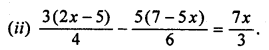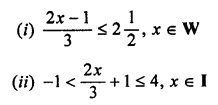## ML Aggarwal Class 7 Solutions for ICSE Maths Chapter 9 Linear Equations and Inequalities Check Your Progress

Question 1.
Solve the following equations :
(i) 2 (x – 5) + 3 (x – 2) = 8 + 7 (x – 4)Solution:
(i) 2 (x – 5) + 3 (x – 2) = 8 + 7 (x – 4)
Removing group symbols,
2x – 10 + 3x – 6 = 8 + 7x – 28
⇒ 5x- 16 = 7x – 20
⇒ 5x – 7x = – 20 + 16
(Transposing -16 to R.H.S and 7x to L.H.S)
⇒ -2x = -4
⇒ x = 2Question 2.
A number exceeds its three-fifth by 22. Find the number.
Solution:
Let the number be x
Three-fifth of a number = $$\frac { 3 }{ 5 }$$ x
According to statement,Question 3.
When 9 is added to twice a number, the result is 3 more than thrice the number. Find the number.
Solution:
Let the number be x
Twice a number = 2x
Thrice a number = 3x
According to statement,
9 + 2x = 3 + 3x
⇒ 9 – 3 = 3x – 2x
⇒ 6 = x
⇒ x = 6

Question 4.
The ten’s digit of a two digit number is twice the unit’s digit. The sum of the number and its unit’s digit is 66. Find the number.
Solution:
Let the unit’s digit be x.
Ten’s digit = 2x.
The number =10 × 2x + x = 20x + x
According to statement,
10 × 2x + x + x = 66
⇒ 20x + x + x = 66
⇒ 22x = 66
⇒ x = 3
The number = 20x + x = 20 × 3 + 3 = 60 + 3 = 63

Question 5.
A student bought some pens at ₹ 8 each and some pencils at ₹ 1.50 each. If the total number of pens and pencils purchased is 16 and their total cost is ₹ 50, how many pens did he buy?
Solution:
The total number of pens and pencils purchased = 16
Let the number of pens bought = x
The number of pencils bought = 16 – x
Cost of pens bought = 8x
Cost of pencils bought = 1.50 (16 – x)
According to given condition,
8x + 1.50 (16 – x) = ₹ 50
⇒ 8x + 24 – 1.50x = 50
⇒ 6.50x = 50 – 24
⇒ x = $$\frac { 26 }{ 650 }$$ × 100 = 4
No. of pens bought = 4

Question 6.
Arvind is eight years older than his sister. In three years, he will be twice as old as his sister. How old are they now?
Solution:
Let the sister’s age = x years
Arvind’s age = (x + 8) years.
In three years, sister’s age = (x + 3) years
In three years, Arvind’s age = (x + 8 + 3) years
According to statement,
x + 8 + 3 = 2(x + 3)
⇒ x + 11 = 2x + 6
⇒ x – 2x = 6 – 11
⇒ -x = -5
⇒ x = 5.
Sister’s age = 5 years
Arvind’s age = 5 + 8 = 13 years.

Question 7.
The angles of a triangle are in the ratio 1 : 2 : 3. Find their measure in degrees.
Solution:
Let the angles of a Δ are x, 2x and 3x.
We know that
Sum of ∠s of a Δ is 180°
x + 2x + 3x = 180°
⇒ 6x = 180°
⇒ x = 30°
∠s of a Δ are
1x = 1 × 30° = 30°,
2x = 2 × 30° = 60°,
and 3x = 3 × 30° = 90°

Question 8.
Solve the following inequations and represent their solution on a number line:Solution: